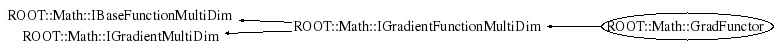```
Documentation for class Functor class.
It is used to wrap in a very simple and convenient way multi-dimensional function objects.
It can wrap all the following types:
<ul>
<li> any C++ callable object implemention double operator()( const double *  )
<li> a free C function of type double ()(double * )
<li> a member function with the correct signature like Foo::Eval(const double * ).
In this case one pass the object pointer and a pointer to the member function (&Foo::Eval)
</ul>
The function dimension is required when constructing the functor.

@ingroup  CppFunctions

```

## Function Members (Methods)

public:
private:
 virtual double DoDerivative(const double* x, unsigned int icoord) const virtual double DoEval(const double* x) const

## Data Members

private:
 auto_ptr fImpl pointer to base grad functor handler

## Class Charts## Function documentation

BaseFunc * Clone()
``` clone of the function handler (use copy-ctor)
```
unsigned int NDim()
``` constructor for multi-dimensional functions
```
double DoEval(const double* x) const
double DoDerivative(double x)
```Default constructor

```
`{}`
GradFunctor( const Func & f, unsigned int dim )
```construct from a callable object of multi-dimension
implementing operator()(const double *x) and
Derivative(const double * x,icoord)

```
`{}`
```construct from a pointer to member function and member function types for function and derivative evaluations

```
`{}`
GradFunctor(void* p1, void* p2, unsigned int dim)
```construct for Gradient Functions of multi-dimension
Func gives the function evaluatiion, GradFunc the partial derivatives
The function dimension is  required

```
`{ }`
```Destructor (no operations)
`{}`